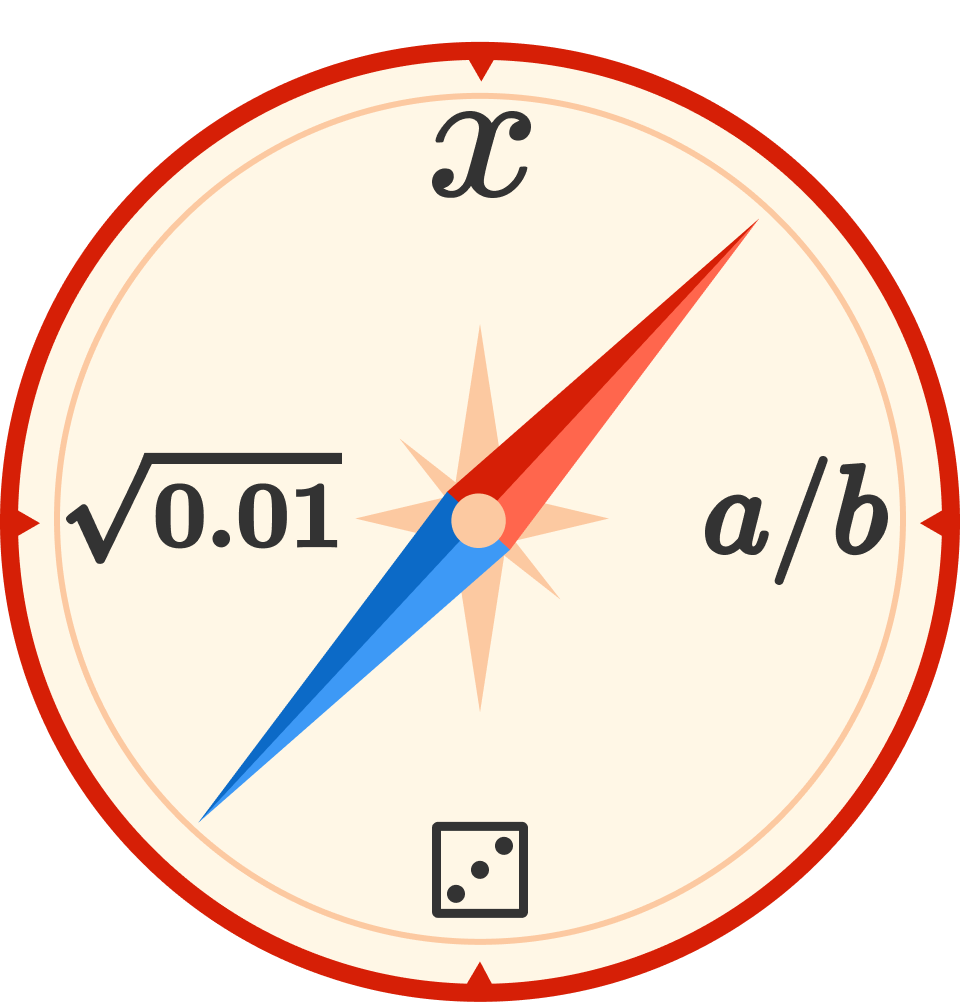# Challenge Set

This course explores introductory logic, algebra, and probability. In this quiz, we'll start with one warmup problem for each of these topics and then challenge you with one trickier problem for each topic.

As you work through the quiz, make sure you look at the solutions. Even if you got a problem correct, it’s a valuable pause to think about the techniques and intuition that you apply in each problem!

## Challenge Set

### Introduction

# Challenge Set

Logic Warmup

You come across a mysterious stoppered flask.You know it contains either a powerful invisibility potion or poison, but you're not sure which. Below is the sign, which you know is false: $\begin{array}{|l|} \hline {\text{It's untrue that this is not poison.}} \\ \hline \end{array}$ Remember, the sign is false. What's in the flask?

## Challenge Set

### Introduction

# Challenge Set

Algebra Warmup

Your favorite store is having a $$50\%$$-off storewide sale! You have a coupon for an additional $$25\%$$ discount.Which option will give you a lower price on a \$200 purchase?

• Option A: The store clerk applies a discount of $$75\%,$$ since that's the sum of $$25\%$$ and $$50\%.$$
• Option B: The store clerk first applies the $$50\%$$ discount, and then applies the $$25\%$$ discount to that value.

## Challenge Set

### Introduction

# Challenge Set

Probability Warmup

All of the sections of Spinner A are the same size, and all of the sections of Spinner B are the same size. Are you more likely to spin green on Spinner A or Spinner B?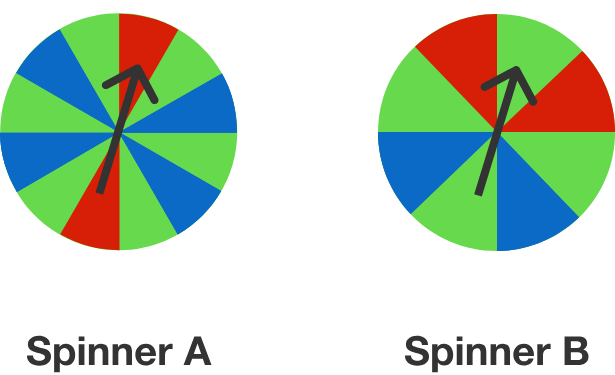## Challenge Set

### Introduction

# Challenge Set

The next three problems will be more difficult. Our philosophy at Brilliant is that you only learn deeply when you're challenged to think for yourself and solve hard problems!

If you can’t figure one out, that’s fine! Just take a look at the solution so that you can learn what technique to use in the future.

While we will cover some traditional logic, algebra, and probability, the problems in this course are also designed to push your mathematical, strategic thinking to the next level!

## Challenge Set

### Introduction

# Challenge Set

Logic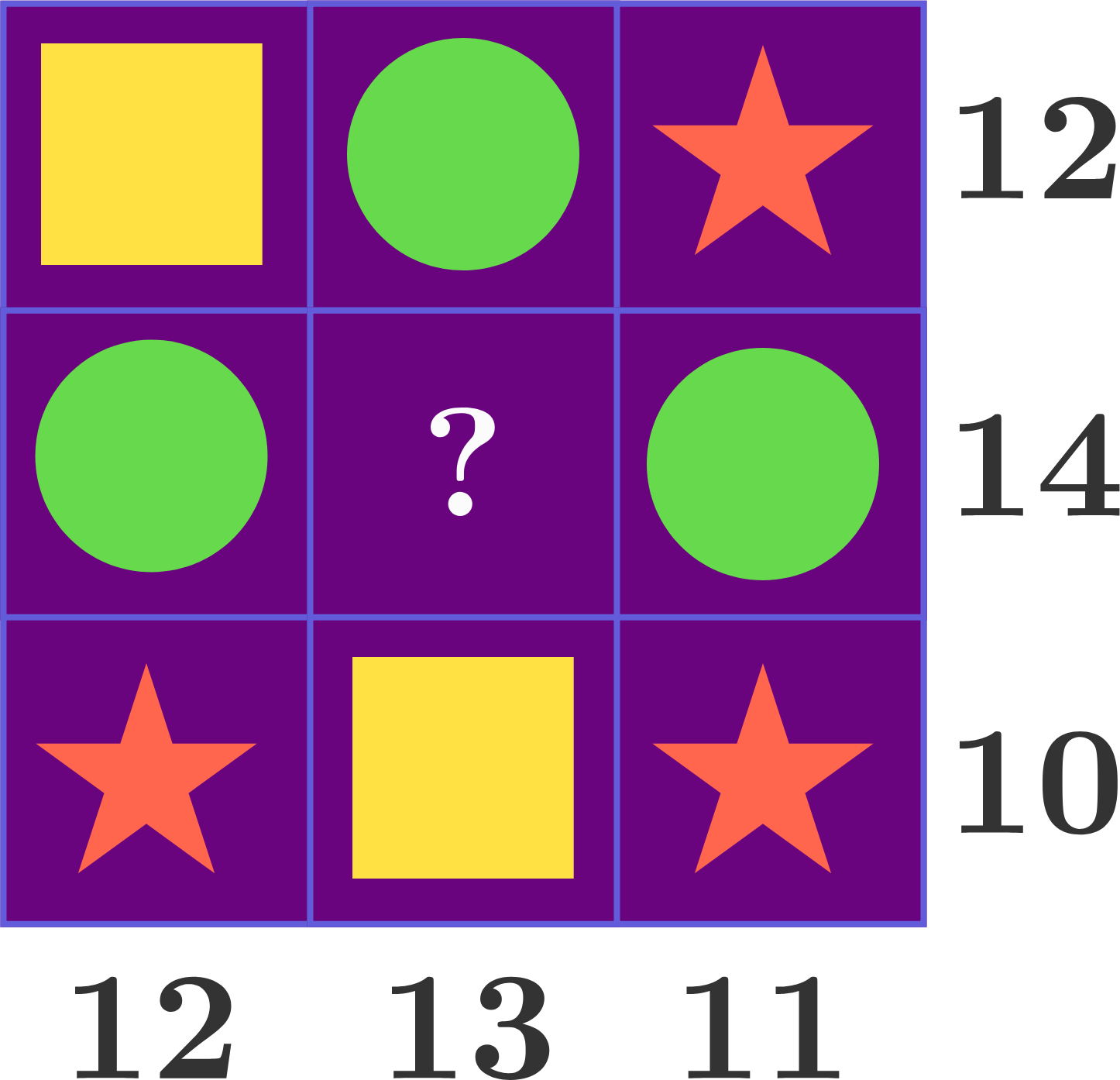Each shape in this puzzle represents a positive integer. The number next to each row or column represents the sum of all of the values in that row or column. Can the center of the grid be filled in with one of the three shapes? If so, which one?

## Challenge Set

### Introduction

# Challenge Set

Algebra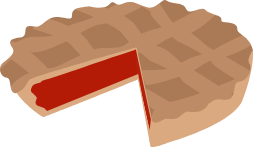It takes 5 cooks 5 hours to bake 5 pies. Assuming no change of rate, how many hours will it take 10 cooks to bake 10 pies?

## Challenge Set

### Introduction

# Challenge Set

Probability

In this game, there are two winning positions (colors), and they are directly next to each other.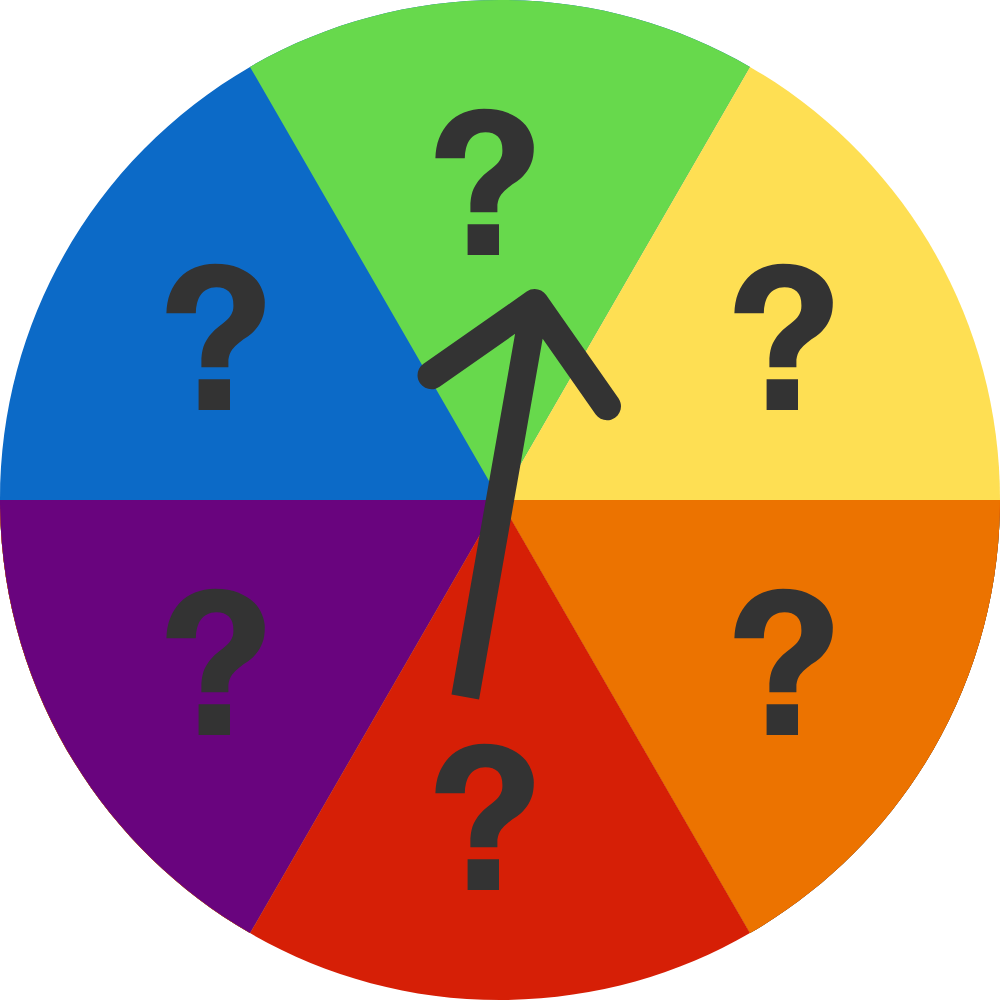A player spins the wheel and it lands on green. They are told that this is not one of the two winning positions, and the next player is then offered a choice:

1) spin the wheel again, where it will land randomly on 1 of the 6 colors, or
2) move the spinner to yellow, the space next to green.

Which choice makes it more likely for the player to land on a winning position?

## Challenge Set

### Introduction

# Challenge Set

For the three problems we solved so far, we focused on:

• trusting our intuition.

• finding a quick strategy for organizing our thoughts.

• simplifying the problem until it seemed easy to find an answer we were confident in.

These are the skills that the problems on Brilliant help strengthen because building up our intuition and general skill set is far more important than memorizing any formula!

## Challenge Set

### Introduction

×